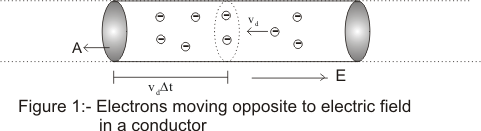Click to Chat

1800-1023-196

+91-120-4616500

CART 0

• 0

MY CART (5)

Use Coupon: CART20 and get 20% off on all online Study Material

ITEM
DETAILS
MRP
DISCOUNT
FINAL PRICE
Total Price: Rs.

There are no items in this cart.
Continue Shopping• Complete Physics Course - Class 11
• OFFERED PRICE: Rs. 2,968
• View Details

```Drift Velocity

The average velocity attained by some particle such as an electron due to the influence of an electric field is termed as the drift velocity. The movement or the motion of the particles is assumed to be along a plane and hence the motion can also be referred to as the axial drift velocity.

The concept of drift velocity can also be understood by studying or noticing the random motion of free electrons moving around in the conductor. These free electrons keep on moving inside the conductor in a haphazard way with random velocities. At times, a conductor may be subjected to an electric field and as a result of it, some kind of electrical force is exerted on the randomly moving electrons but in the direction of the field. This field forces the electrons to switch towards high potential while maintaining their randomness of motion. Scientifically, we can say that the electrons will drift towards higher potentials with their random motions. Hence, we have seen that every electron will have certain net velocity with which it will move towards the higher potential end of the conductor. This net velocity is termed as the drift velocity of the electrons. Since the electrons are said to be moving with drift velocity, even the current which results due to this drift movement of electrons in an electrically charged conductor is called as the drift current. In fact, every current flowing through the conductor is termed as the drift current.

When the charged particles move around in a conductor, their motion is not in a straight line because of their collision with other particles in the conductor. Therefore, the average speed of the particle in the conductor is taken into consideration. This is termed as the drift velocity.

Formula to calculate Drift Velocity using Current Density

Formula for calculating drift velocity of electron in a conductor of constant cross-sectional area is given by

V = 1/ nAq

Where, ‘v’ is the drift velocity of the electrons

‘I’ is the current flowing through the conductor (amperes)

‘A’ is the cross-section area of the conductor (m2)

‘q’ is the charge on the charge carrier (coulombs, c)

Example of Drift Velocity

So, the above formula of drift velocity clearly illustrates that if any of the three quantities out of the four in the formula are given, the missing quantity can be found out easily. Hence, the drift velocity of electrons in a particular piece of metal exhibiting a current of 0.1 A will be around 1x10-5 m/s. Generally, the electrons move at very high speeds of around a million m/s along a wire but they drift or move very slowly in the direction of the current.

What is the relation between Drift Velocity and Electric Current?

We take a conducting wire of length L exhibiting a uniform cross-section area ‘A’ which contains electric field. Let us assume that this wire contains ‘n’ free electrons per unit volume each possessing a drift velocity ‘v’. If we consider a small time interval ?t, then the distance covered by each electron in this time interval is v?t. The volume of this specific portion of area is given by Av?t. There are a number of free electrons in this portion amounting to nAv?t and they pass through the cross-section A in time ?t.If we denote the charge with ?Q, then the charge crossing the area in time ?t is given by

?Q = ne Av?t

Or it can be rewritten as I = ?Q / ?t = neAv

This is the equation that describes the relation between drift velocity and electric current.

Moreover, if the charge carriers in motion are positive and not negative then the field force acting on the carriers would be in accordance with the direction of electric fields and hence, the drift velocity would be from left to right. There is also a relation between the current density and the drift velocity which is described by the equation

J = I/A = nev

You may watch this video for more on drift velocity

askIITians offers comprehensive study material which covers all the important topics of IIT JEE Physics. The content is perfectly designed for the JEE aspirants keeping in mind the requisites of the JEE. All the areas like the drift velocity formula along with the example for drift velocity have also been included in the content. It is a must to have a good hold on this topic in order to remain competitive in the JEE.

Related Resources

Look into the Sample Papers with Solutions to get a hint of the kinds of questions asked in the exam.

You can get the knowledge of Important Books of Physics here.

To read more, Buy study materials of Current Electricity comprising study notes, revision notes, video lectures, previous year solved questions etc. Also browse for more study materials on Physics here.
```### Course Features

• 101 Video Lectures
• Revision Notes
• Previous Year Papers
• Mind Map
• Study Planner
• NCERT Solutions
• Discussion Forum
• Test paper with Video Solution